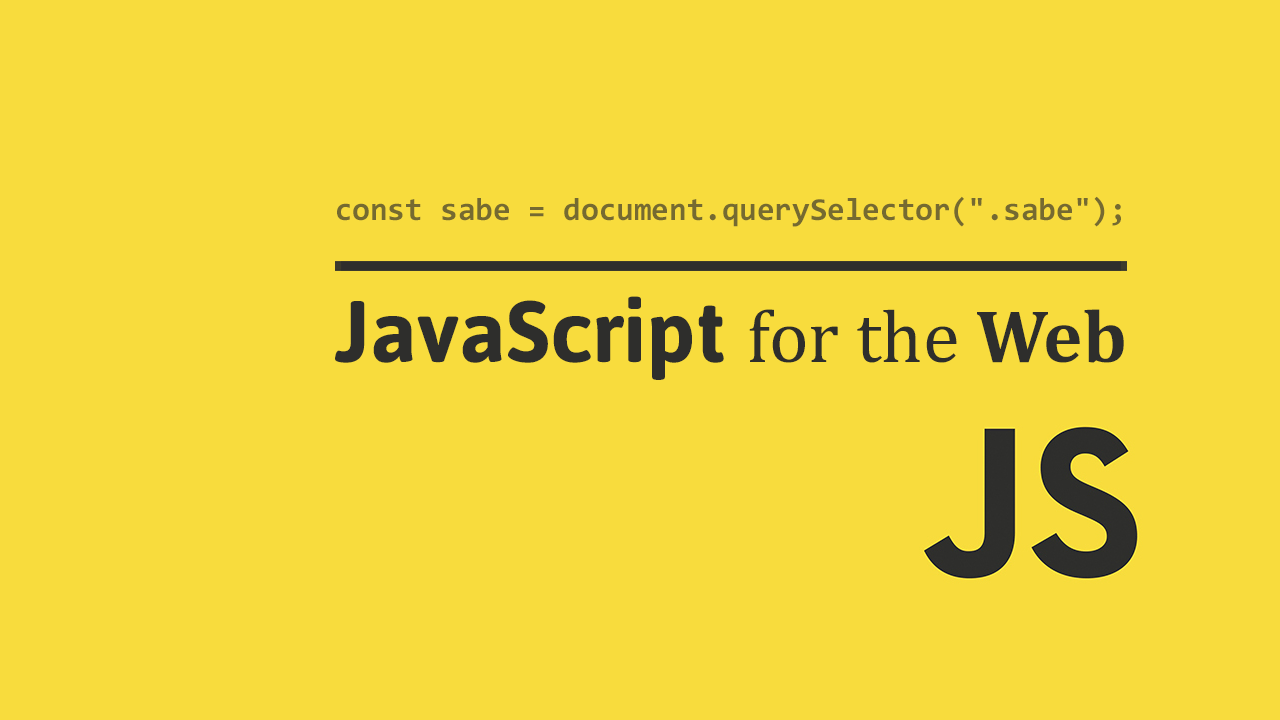## Functions

Functions are blocks of code that can be reused over and over again. By creating a function, you can eliminate the need to write the code multiple times, and it helps your programs become more modular and easier to work with.

You can divide up larger tasks into smaller chunks so that your code doesn't grow out of control. Let's start with a simple function, say you want to display an introduction.

```	```
function introduction() {
console.log("Hello, my name is Jessica.");
}
```
```

To create a function, use the `function` keyword, followed by the name of the function. In our example, the name is of this function is `introduction`.

Another way to create a function is to use the arrow function syntax. Here is the same function using the newer syntax.

```	```
const introduction = () => {
console.log("Hello, my name is Jessica.");
};
```
```

In this case, we have actually set the function to a new `const` instead.

## Invoking

Now that we have created a function, we now need to invoke the function, also known as calling the function. In other words, we want to use it.

So let's use it!

```	```
function introduction() {
console.log("Hello, my name is Jessica.");
}

introduction();
```
```
```	```
Hello, my name is Jessica.
```
```

There you have it, you invoked the `introduction` function successfully! What if, however, your name isn't Jessica, and you want to be able to pass in both a custom greeting and your own name?

## Parameters

You can pass in data to your functions. These pieces of data are called parameters, or arguments.

Let's pass a custom greeting and name to our `introduction` function, and then invoke it.

```	```
function introduction(greeting, name) {
console.log(greeting + ", my name is " + name + ".");
}

introduction("Hi", "Nabeed");
```
```
```	```
Hi, my name is Nabeed.
```
```

Our new `introduction` function now takes a greeting and name, and concatenates it with other strings to make a new introduction that works with anybody!

## Return Values

Functions can return data to us. In our `introduction` function, there was nothing to return because we just printed some text and that was it.

Let's say instead we want to write a function that returns the area of a circle by providing it with the radius as a parameter.

```	```
}

console.log("A circle with a radius of " + radius + " has an area of " + area + ".");
```
```

Our `getAreaOfCircle` function has the radius as its only parameter. It takes it, squares it by multiplying it by itself, then multiplies the whole thing by the value of Pi.

The function then returns that value, which is assigned to the `area` variable here:

```	```
Now with the value of `radius` being `3` and the value of `area` being `28.274333882308138`, we can now print out the values of both variables.
```	```